info
Back to overview

# 6 Time-Saving Baserow Formulas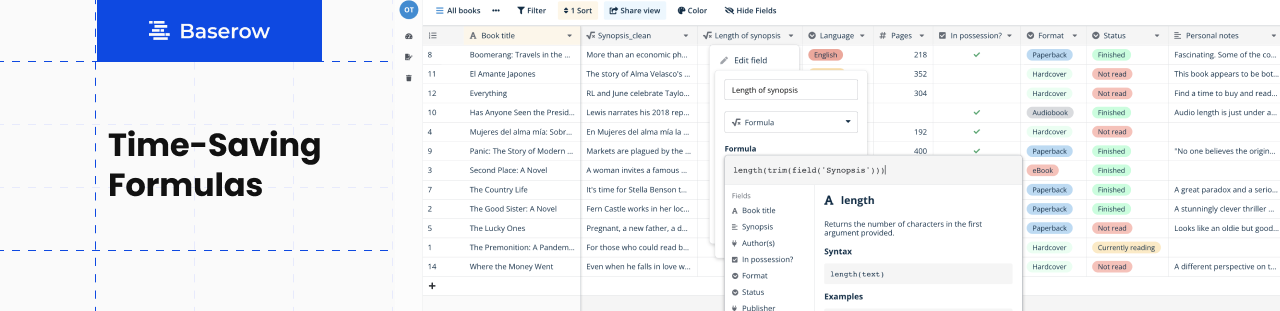Are you getting all you can out of Baserow?

We compiled a list of six simple yet effective formulas that you can use to save time, improve your workflows, and simplify data analysis. Check out the overview of these functions to max up your data management.

## 1. Function: Length

The Length function counts the number of characters in a cell.

``````length(text)
``````

In a table used to track blog posts, for example, this is an extremely useful formula to check the number of characters in an article or meta description. You can use a formula like the following to output the character count of the text field:

``````length(field('Meta description'))
``````

Pro tip: use the IF function to display a message based on character count.

Naturally, one of the most popular uses for the formula is detecting how many characters you have put in, and if you have exceeded the specified limit. A simple IF statement can be used to display an output message based on the number of characters.

``````if(field('Meta description length')<=160, '✅', '❌')
``````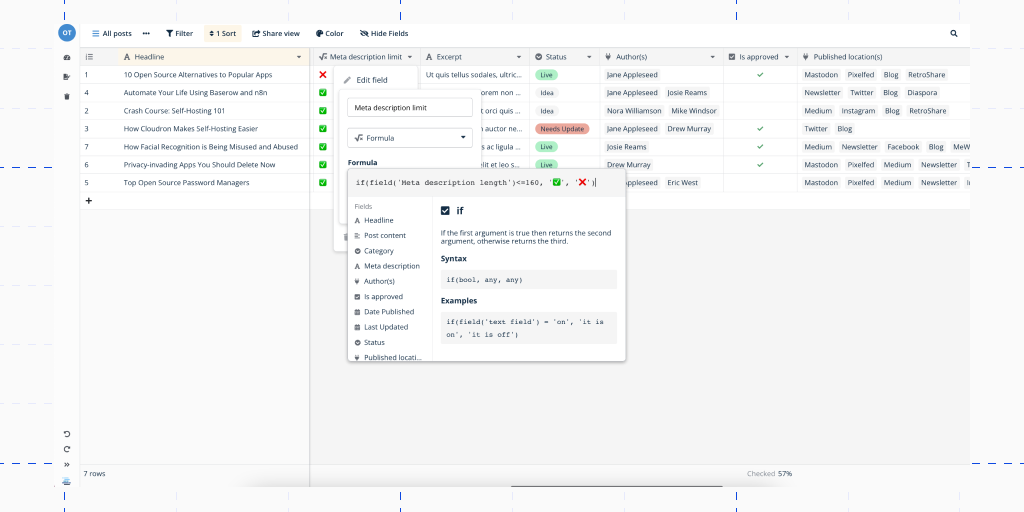## 2. Function: Concat

The Concat function combines arguments and/or field values from multiple fields into a single piece of text.

``````concat(any, any, ...)
``````

Note: Use `" "` as a separator between the fields.

One of its most popular uses is to combine several values together in the primary field to have better visibility of the most important information in the first column.

We will use a CRM system as an example. It may be helpful to use the primary field to see the candidate’s name and the stage of the sales process. Remember, the data will be updated due to the latest changes in both of the linked fields.

``````concat(field('Company'), " - ", field('Stage'))
``````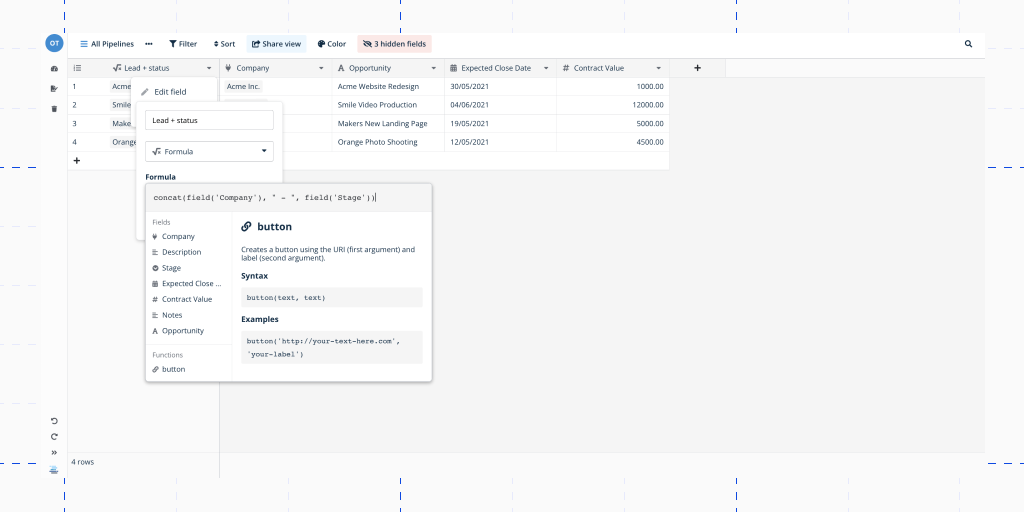## 3. Function: Date diff

The `Date diff` function calculates the difference between two dates.

Finding the difference between the two dates can be helpful for many workflows. Using the `date_diff()` function in the example below, we will find the difference in days between two specified dates.

``````date_diff(text, date, date)
``````

Note: In your formulas, the end date must always be greater than the start date, otherwise the Date Diff function returns an error.

One of the use cases for this function is to calculate how many days it took for a payment to be processed: one date is when the invoice was sent, and the other is when the payment was received.

``````date_diff("day", field('Transaction date'), field('Invoice day'))
``````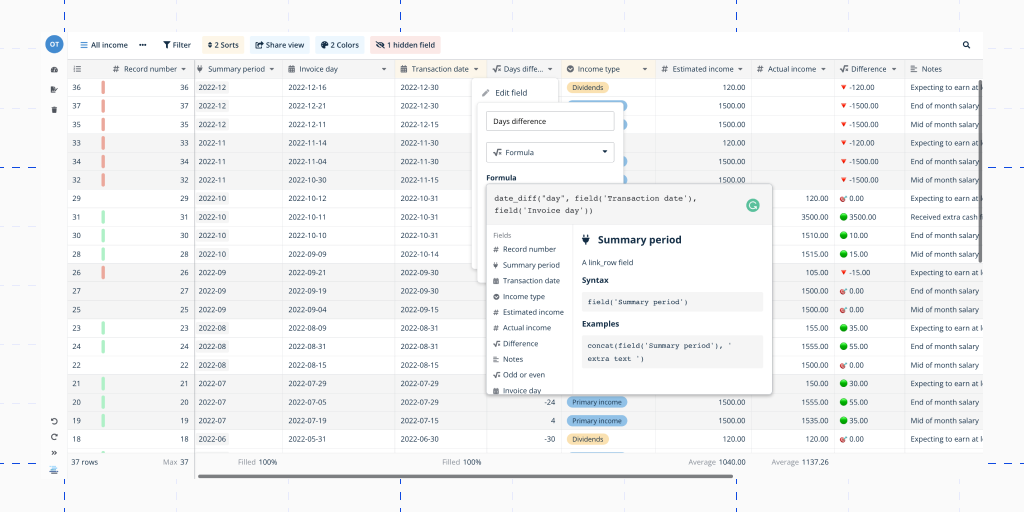Note: In Baserow you can calculate the difference in ‘year’, ‘month’, ‘week’, ‘day’, ‘hour’, ‘minute’, and ‘seconds’.

### Alternative to `date diff`

We can also use the minus operator to subtract two arguments.

The same function carries the formula with an ampersand `(-)` as a minus character between values. See how we rewrote the formula from the example above using this alternative:

``````field('Transaction date') - field('Invoice day')
``````

## 4. Function: Trim

The `TRIM` function removes spaces from the beginning and end of a string.

``````trim(text)
``````

The results will include the contents of the text field in each record without blank spaces at the beginning and end of the text.

You can find a use for it when copy-pasting extracts from the Internet, and you don’t want to have any extra spaces at the beginning and the end of a text. For instance, when adding a book synopsis to your reading table.

``````trim(field('Synopsis'))
``````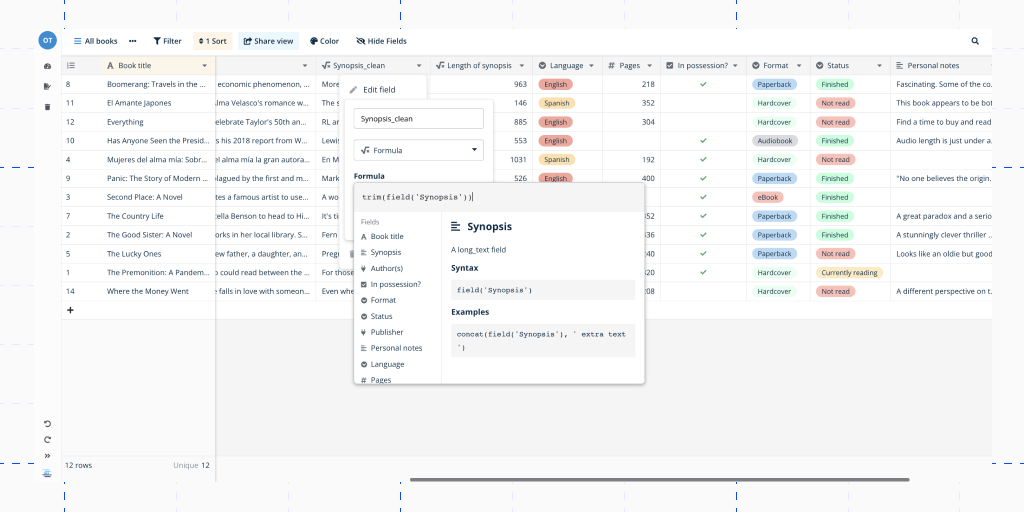Pro tip: Combined with other functions, TRIM can help you accomplish more.

For example, if you want the character count formula to not count blank spaces at the beginning and/or end, use the following string:

``````length(trim(field('Synopsis')))
``````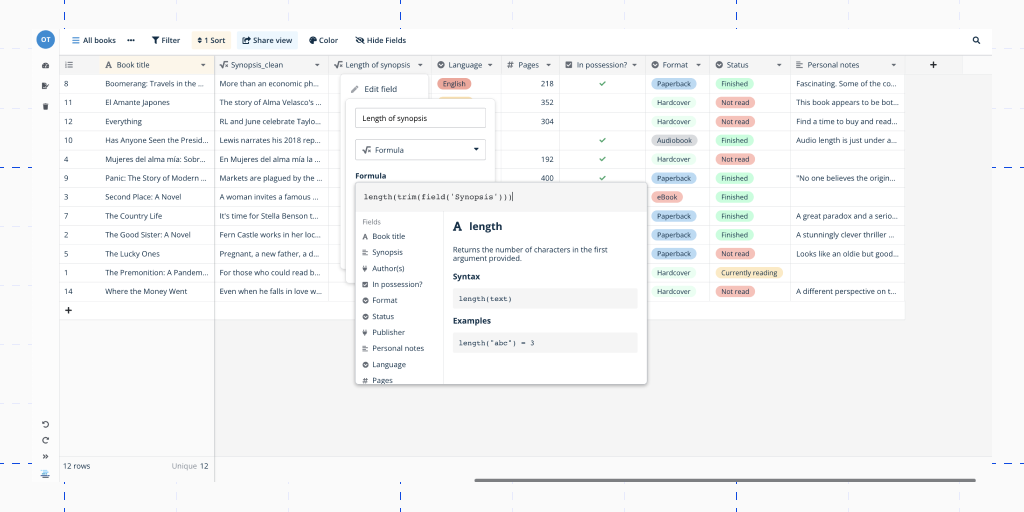## 5. Function: Left/right

The `left()` and `right()` functions are used to extract a certain number of characters from the beginning (left) or end (right) of a string.

``````right(text, number)
left(text, number)
``````
• `left("you are the best community member", 5)` would result in “Community”
• `right("you are the best community member", 3)` would result in “Best”

These are helpful functions to use if you need to extract data from a number of records that share a similar structure. For example, you may be importing a dataset where a single cell contains both a date string and a text string. These functions also support negative numbers.

Assuming that the dates follow the same format as DD-MM-YYYY, you can use `right()` to extract the date from the full field value:

``````right(totext(field('Date & time')), 6)
``````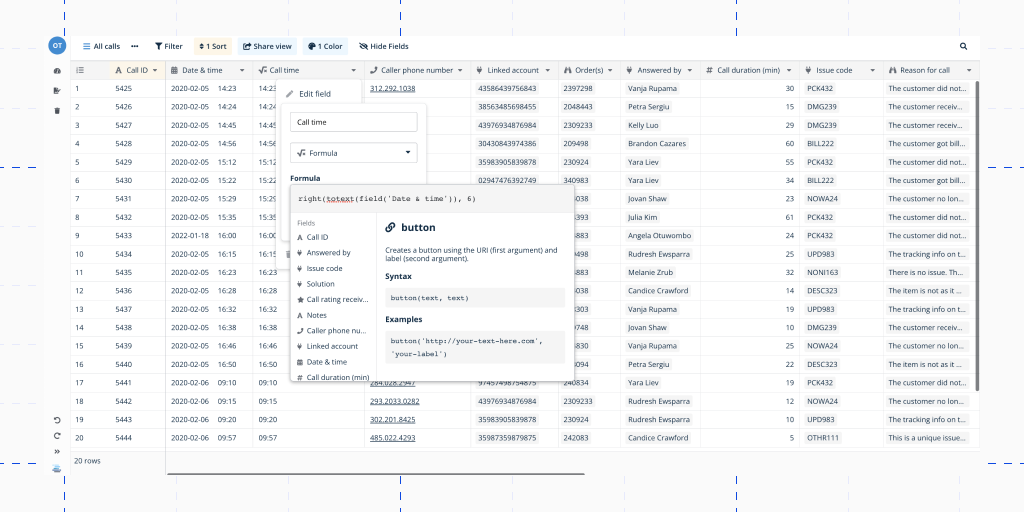## 6. Function: Upper/lower

The `lower` function takes a string of text and makes all the characters lowercase. Conversely, `upper` changes all characters in a string of text to uppercase. On their own, these functions can help you ensure text is kept in the appropriate case.

Their functionality is very simple: `lower("The Weather is Very Good Today.")` results in the `weather is very good today`. Replacing `lower` with `upper` accomplishes the opposite result: `THE WEATHER IS VERY GOOD TODAY.` Let’s see how this function works. Imagine, you need to uppercase the first letter in a word:

``````upper(left(trim(field("Text")),1))+right(trim(field("Text")), length(trim(field("Text"))) - 1)
``````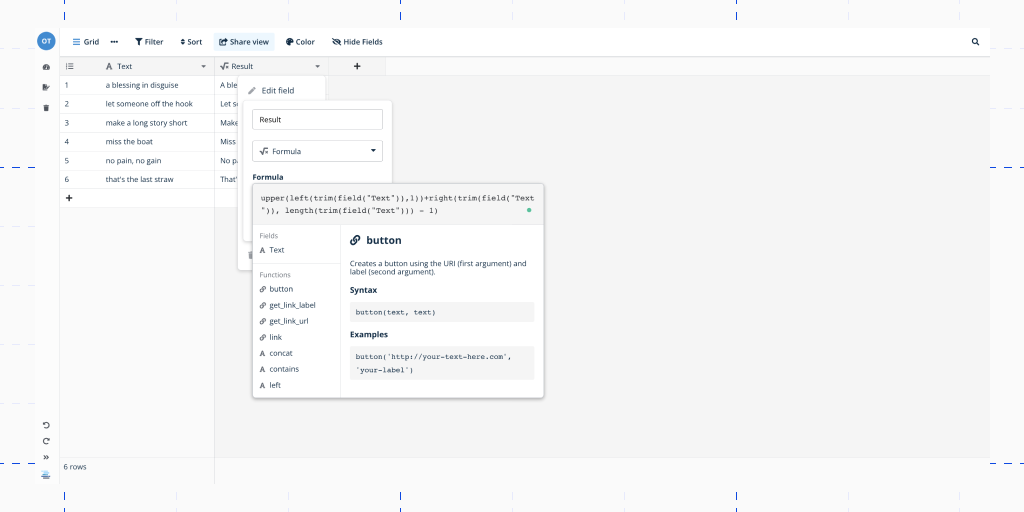Use these powerful formulas to take control of your data and open up new possibilities in your workflows.

## Have another formula in mind?💡

If you have a great formula in mind or a way to substitute the ones we showed you — do not hesitate to drop a message in the community forum!

Also, if you can’t seem to figure out which formula to use to perform a specific function, post your question in the forum and we are sure some community members will come to your help!

release
May 16, 2023 by Bram Wiepjes
Release 1.17: up to 3x faster

Today we are releasing Baserow 1.17 with huge performance improvements for high volumes of data, calendar view, ARM images & more…!

info
January 26, 2023 by Georgina Brisk
Best Airtable alternatives
info
January 10, 2023 by Olivier Maes
No-Code Platforms in 2023
Want to contribute?

Want to write a post in collaboration with us?